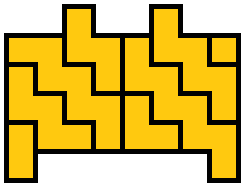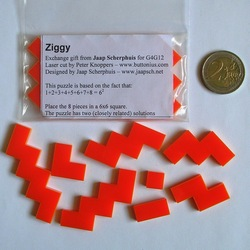Ziggy is a small packing puzzle that I designed. I used it as the exchange gift for the Gathering for Gardner 12 conference in 2016.

The puzzle is based on the fact that 1+2+3+4+5+6+7+8 = 62. There are 8 zig-zag polyomino pieces, ranging in size from 1 to 8 squares. The basic aim is to create a 6 by 6 square. The pieces are shown here: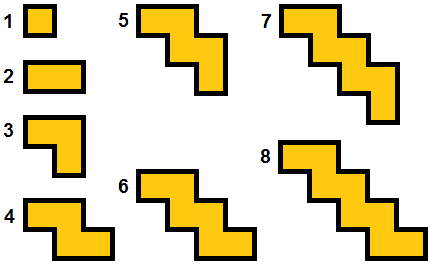There are two very closely related solutions to the square, but there are also several other challenges you may like to try. Here is a selection of 18 shapes that can be made from the 8 pieces:

1.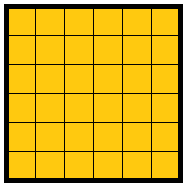2.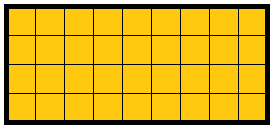3.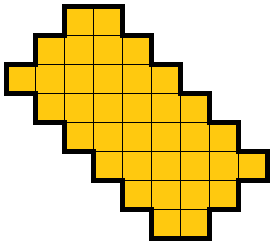4.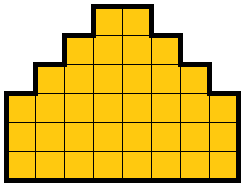5.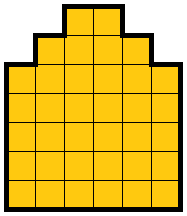6.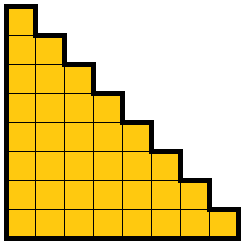7.8.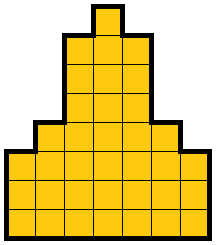9.10.11.12.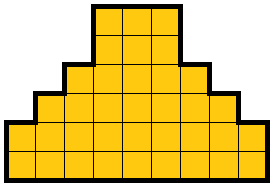13.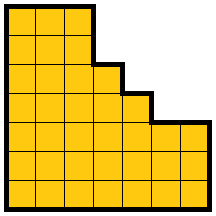14.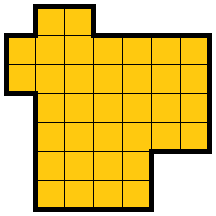15.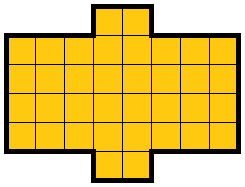16.17.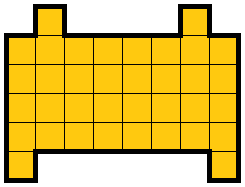18.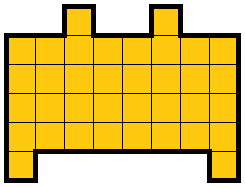## Solutions

Here are solutions to each of the challenges. Both solutions to the 6 by 6 square are given, but for the other challenges only one solution is shown even though there may be more.

1a.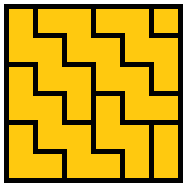1b.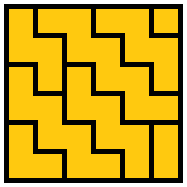2.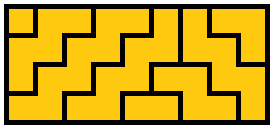3.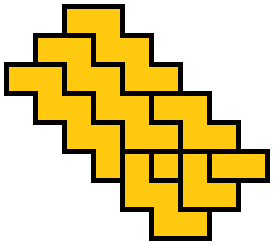4.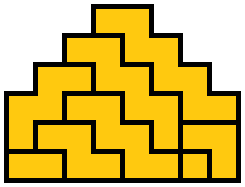5.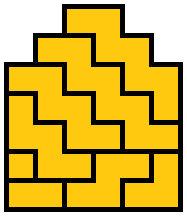6.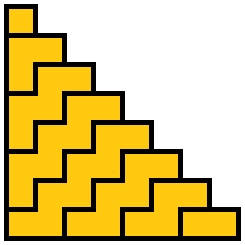7.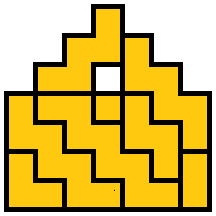8.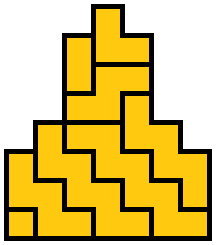9.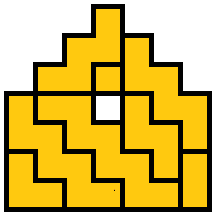10.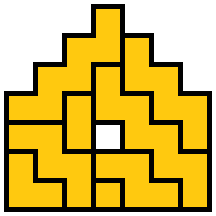11.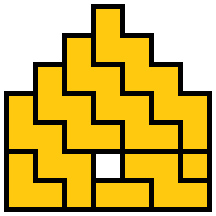12.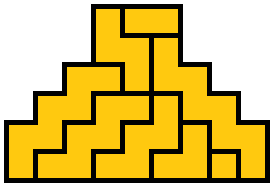13.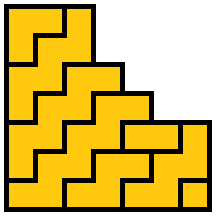14.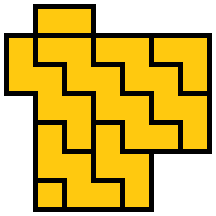15.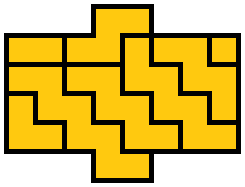16.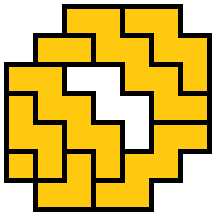17.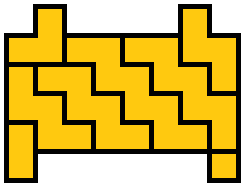18.